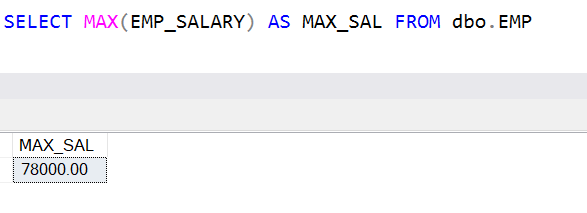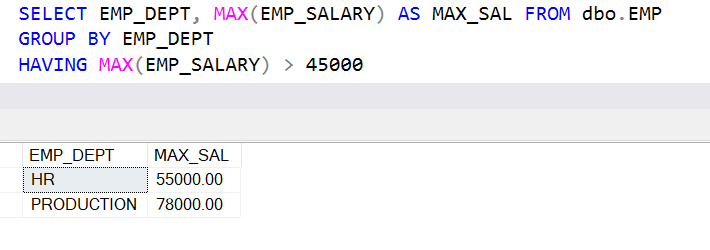Home » SQL Server MAX

# SQL Server MAXSQL Server MAX()  function is an aggregate function that is used to find the Maximum or Largest value of a column.

If a table have only single value then the maximum value will be a value itself.

If all values in a table are same then the maximum value will be a value itself.

Syntax

`MAX ( columnname )`

Lets look at an example of MAX() function in SQL Server.

Here we have a EMPLOYEE table named as EMP.

```CREATE TABLE dbo.EMP (
EMPID INT NOT NULL,
EMP_DEPT VARCHAR(50),
EMP_NAME VARCHAR(50),
EMP_SALARY NUMERIC(9,2)
)

INSERT INTO dbo.EMP
(EMPID, EMP_DEPT, EMP_NAME, EMP_SALARY)
VALUES
(101, 'PRODUCTION', 'RAJAT M',75000.00),
(102, 'PRODUCTION', 'MUKESH BHATIA',70000.00),
(103, 'PRODUCTION', 'MUSKAN MEHTA',75000.00),
(104, 'SALES', 'ROHAN B NARAYA',45000.00),
(105, 'SALES', 'SUSHIL DAS',40000.00),
(106, 'SALES', 'MANISH',45000.00),
(107, 'PRODUCTION', 'RAJESH SINGH',78000.00),
(108, 'HR', 'MOHIN KHAN',50000.00),
(109, 'HR', 'SUSHANT K SINGH',55000.00),
(110, 'HR', 'LAKSHYA RAWAT',55000.00),
(111, 'PRODUCTION', 'MANOJ KUMAR',75000.00),
(112, 'SALES', 'SUJOY M',40000.00),
(113, 'LOGISTIC', 'VINAY AGARWAL',35000.00),
(114, 'LOGISTIC','MUSTAKIM M',35000.00),
(115, 'LOGISTIC', 'VIJAY KUMAWAT',45000.00)```

To get the Highest salary in the entire table

`SELECT MAX(EMP_SALARY) AS MAX_SAL FROM dbo.EMP`#### Get the highest salary that is given to employees in each DEPARTMENT

```SELECT EMP_DEPT, MAX(EMP_SALARY) AS MAX_SAL FROM dbo.EMP
GROUP BY EMP_DEPT```#### Get the highest paid salary for PRODUCTION Department

```SELECT MAX(EMP_SALARY) AS MAX_SAL FROM dbo.EMP
WHERE EMP_DEPT = 'PRODUCTION'```#### Get the highest paid salary in each department that is greater than 45000

```SELECT EMP_DEPT, MAX(EMP_SALARY) AS MAX_SAL FROM dbo.EMP
GROUP BY EMP_DEPT
HAVING MAX(EMP_SALARY) > 45000```SQL AVG Function

SQL COUNT Function

SQL MIN Function

SQL SUM FunctionThis site uses Akismet to reduce spam. Learn how your comment data is processed.